Browse by Topic
Related Topics

# Visualize a Satellite Path with Wolfram SystemModeler and Mathematica

Explore the contents of this article with a free Wolfram SystemModeler trial.Today we rely heavily on satellites orbiting Earth for a variety of purposes. Mapping satellites are used to collect satellite images used in maps. Communication satellites are used for both telecommunication and internet access or for navigation services like GPS and GLONASS. Other usage areas are weather study, scientific observation, and reconnaissance.

The following model, created in Wolfram SystemModeler, is of a geocentric, inclined circular Low Earth Orbit (LEO) satellite. Geocentric means that it orbits around the Earth. An inclined circular orbit means that the orbit follows a circle, but is not aligned with the equator of the Earth. LEO is the name given to the altitude range below 2,000 kilometers (1,200 miles).

Suppose you are considering using this geocentric LEO satellite to collect image data. To achieve this, you would want to know where it is at the moment, how high it is, and how fast it’s going. If you want images of cities, you want to know over which cities it currently is. A SystemModeler model combined with data and computational resources in Mathematica can answer all of these questions.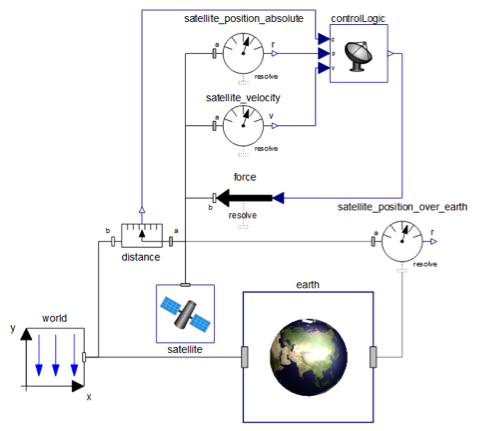Creating such a model is straightforward in SystemModeler. Using drag-and-drop, create three subsystems. Model the Earth using a mass with constant rotation, the satellite using a mass with propulsion forces, and the control logic using two proportional derivative (PD) controllers.

This blog post focuses on illustrating the orbit and flight of the satellite in the above model.

Simulation results from a modeled system can often be hard to grasp and hard to understand without further processing and reasoning. The following plot shows the satellite position in space: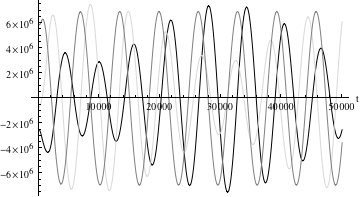The three curves show the position in the directions x, y, and z. This plot alone will not tell you a lot about the satellite. It cannot, for example, answer questions like “Over which part of the globe is the satellite?“, “What is the velocity of the satellite?“, or “What large city is the satellite closest to?” It could answer the question “At what altitude is the satellite?“, but that would require some manual computation.

The goal of this post is to illustrate how Mathematica and SystemModeler can work together to answer the questions above in a visual and clear way.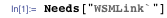Then load and simulate the satellite model for 100,000 seconds: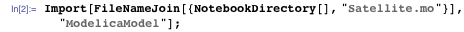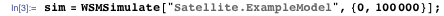Then pick out some data needed for the visualizations from the simulation result object: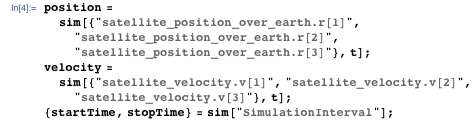As we work in Mathematica, we have access to continuously updated data sources for a variety of things. We are going to use that data for two purposes: retrieving a list of large cities and their coordinates and getting the radius of the Earth. Lets start with the cities, where we use CountryData and CityData: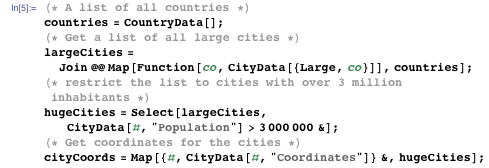The Earth radius is available in AstronomicalData: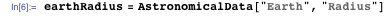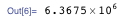In the interest of conciseness, we use a ready-made 3D plot of the Earth. An example of how to create such a plot can be found on the Texture reference page.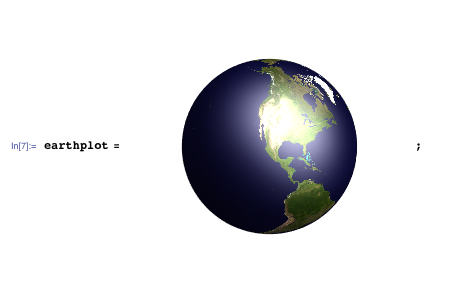Now we are ready to start creating the pieces of our visualization. Start by creating a 3D plot with a point at the satellite’s current position. Define a function that returns that plot: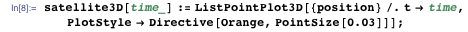Show the position together with the Earth. The ViewVector option defines from which direction the plot is viewed. Also define a variable for the time at which we test all our visualizations: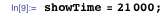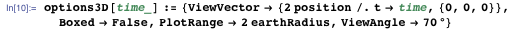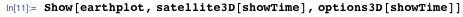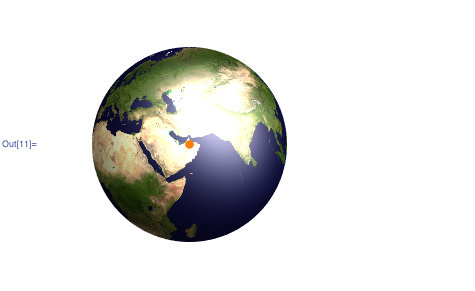The path of the satellite would be clearer if we also plot that path in the same 3D plot. Let’s do that using a 3D parametric plot, again defining a function to calculate it. We also add some color to the path, using the ColorFunction option, and increase the number of PlotPoints to get a smooth look: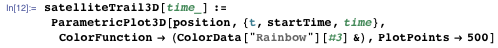Adding this to the previous plot is simple; we just add it to the list of things to show: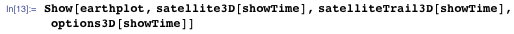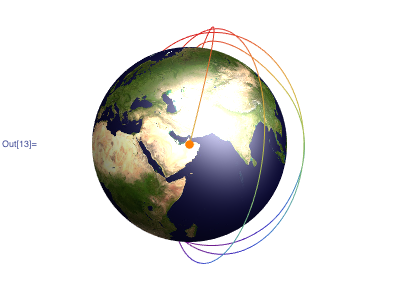That is a nice 3D view of the satellite, and we can see where the satellite is above the Earth at any time. Such a 3D plot makes for a great interactive view, allowing you to rotate and manipulate the display.

Another way to display the path of the satellite is on a 2D map of the world. This is how Wolfram|Alpha displays the current position of the International Space Station.

The function CountryData that we used before also has graphic representations of countries and regions. We use this as our source for a world map. Choose an equirectangular projection: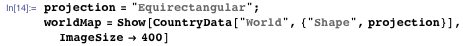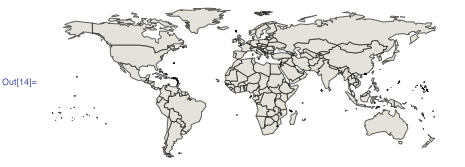Place the satellite and its path on this map using the graphic primitives Disk and Line. Because the equirectangular projection maps directly to longitude and latitude, we can use LatitudeLongitude to pick out the graphics coordinates from a GeoPositionXYZ object: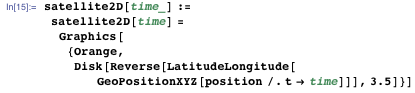We split the position list into multiple lines, starting a new line each time the satellite crosses the edge of the graphic.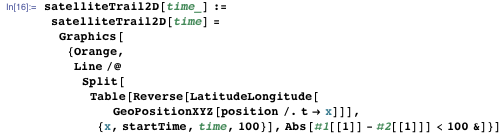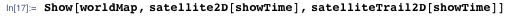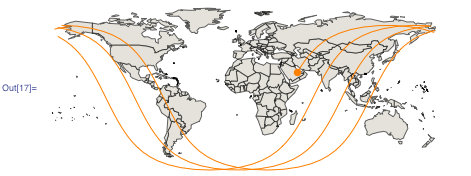Another interesting thing to know is which cities are closest to the satellite. Use the list of cities we retrieved earlier in the blog post. Create a table of all the cities and their distances to the satellite. The distance is calculated from the point directly under the satellite using GeoDistance.

Then sort the table by distance and take the first three cities: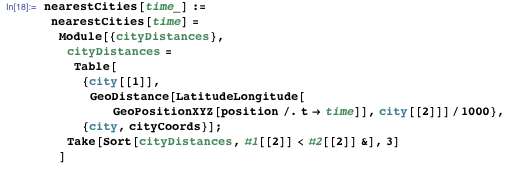Get the nearest cities and show them in a grid: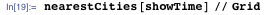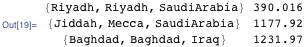Also create functions for the velocity and altitude of the satellite: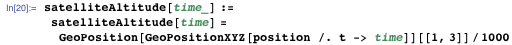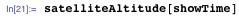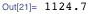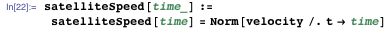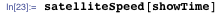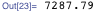Now we have all the visualizations needed to create a nice report: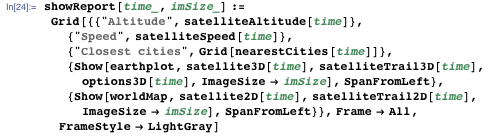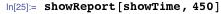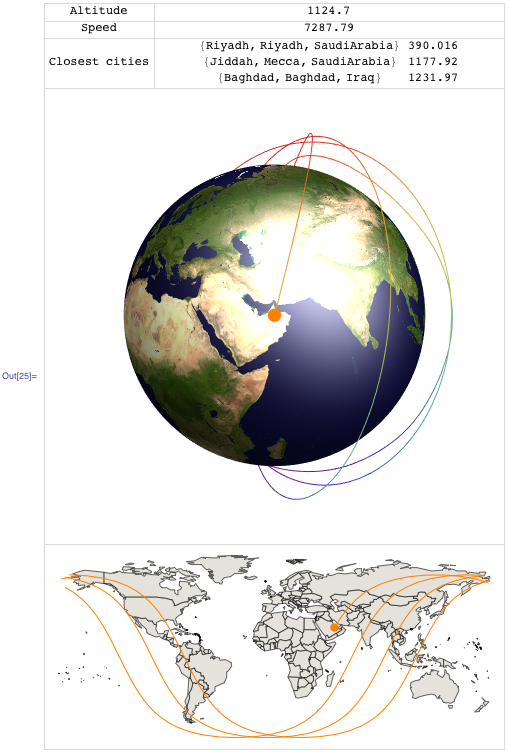A way to further visualize the simulation results and the satellite trajectory is to make an animation over time. This is done in the following video by rearranging into a horizontal layout and exporting as video:

With these visualization functions and the model, which are downloadable below, you can do further analysis, answering and studying a range of different questions. For example, what happens if different altitude controllers are used? How much energy is needed to launch the satellite or to keep it at a stable altitude? What about if a sudden avoidance maneuver is required to avoid space debris or another satellite? How many satellites like this would be required to give “coverage” to the whole Earth or to a certain percentage of the population or area?

Download the full satellite model, including documentation and examples, from the industry examples page and start exploring!